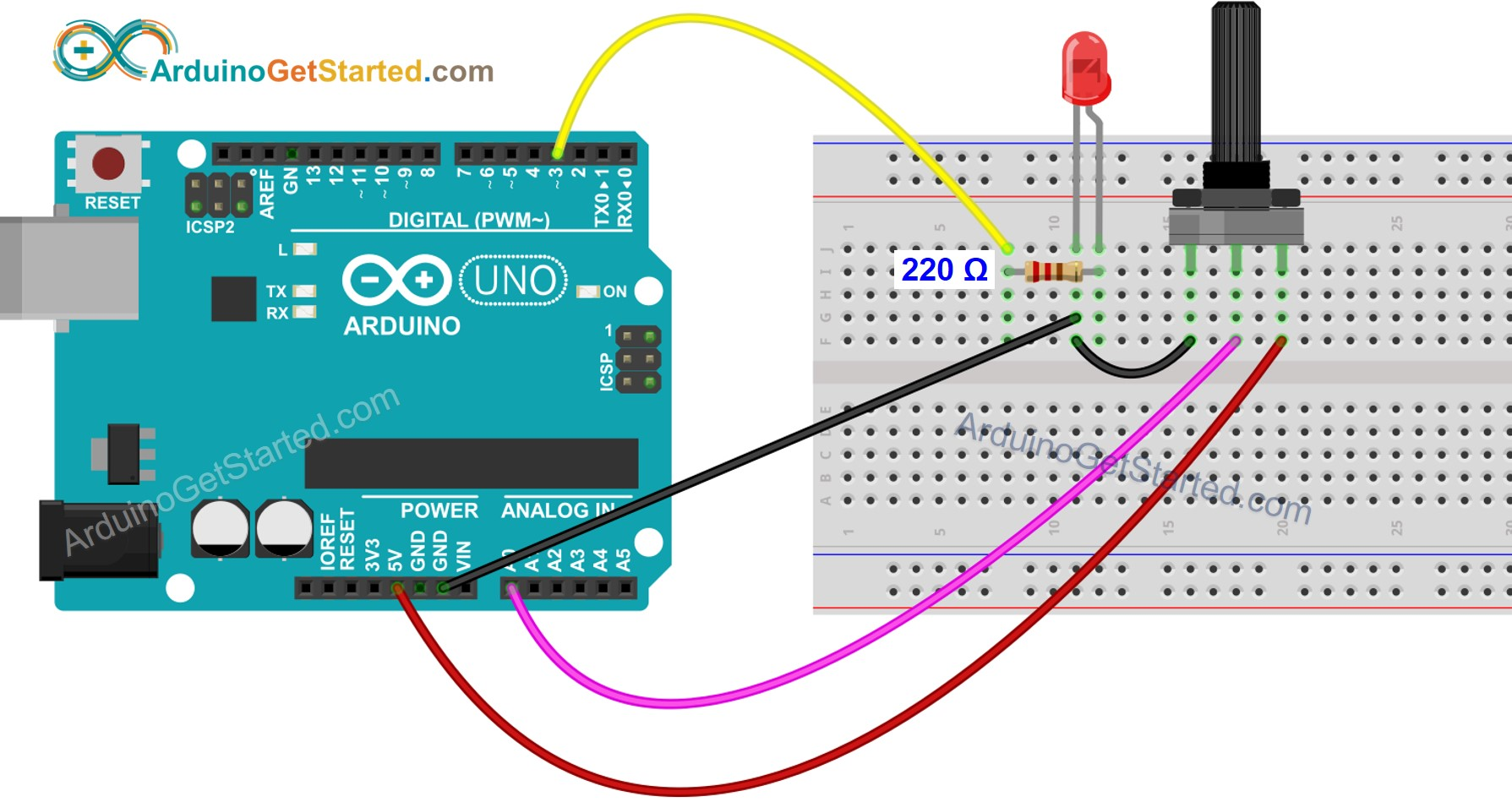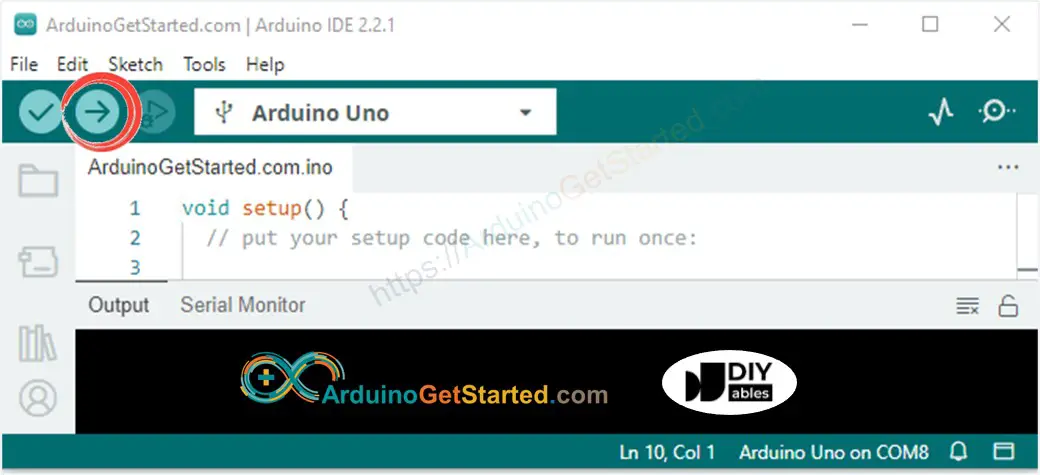# Arduino - Potentiometer Triggers LED

In a previous tutorial, We have learned how to change the brightness of LED according to the potentiometer's output value. In this tutorial, We are going to learn how to:

• If the potentiometer's analog value is greater than a threshold, turn LED on
• If the potentiometer's analog value is lower than a threshold, turn LED off
• If the potentiometer's output voltage is greater than a threshold, turn LED on
• If the potentiometer's output voltage is lower than a threshold, turn LED off

## Hardware Required

Or you can buy the following sensor kit:

Please note: These are Amazon affiliate links. If you buy the components through these links, We will get a commission at no extra cost to you. We appreciate it.

If you do not know about LED and potentiometer (pinout, how it works, how to program ...), learn about them in the following tutorials:

## Wiring DiagramThis image is created using Fritzing. Click to enlarge image

## Arduino Code - Analog Threshold

/* * Created by ArduinoGetStarted.com * * This example code is in the public domain * * Tutorial page: https://arduinogetstarted.com/tutorials/arduino-potentiometer-triggers-led */ // constants won't change const int POTENTIOMETER_PIN = A0; // Arduino pin connected to Potentiometer pin const int LED_PIN = 3; // Arduino pin connected to LED's pin const int ANALOG_THRESHOLD = 500; void setup() { pinMode(LED_PIN, OUTPUT); // set arduino pin to output mode } void loop() { int analogValue = analogRead(POTENTIOMETER_PIN); // read the input on analog pin if(analogValue > ANALOG_THRESHOLD) digitalWrite(LED_PIN, HIGH); // turn on LED else digitalWrite(LED_PIN, LOW); // turn off LED }

### Quick Steps

• Connect Arduino to PC via USB cable
• Open Arduino IDE, select the right board and port
• Copy the above code and open with Arduino IDE
• Click Upload button on Arduino IDE to upload code to Arduino• Rotate the potentiometer
• See the change of LED's state

### Code Explanation

Read the line-by-line explanation in comment lines of source code!

## Arduino Code - Voltage Threshold

Potentiometer's analog value is converted to voltage value, and then compared to voltage threshold to triggers LED

/* * Created by ArduinoGetStarted.com * * This example code is in the public domain * * Tutorial page: https://arduinogetstarted.com/tutorials/arduino-potentiometer-triggers-led */ // constants won't change const int POTENTIOMETER_PIN = A0; // Arduino pin connected to Potentiometer pin const int LED_PIN = 3; // Arduino pin connected to LED's pin const float VOLTAGE_THRESHOLD = 2.5; // Voltages void setup() { pinMode(LED_PIN, OUTPUT); // set arduino pin to output mode } void loop() { int analogValue = analogRead(POTENTIOMETER_PIN); // read the input on analog pin float voltage = floatMap(analogValue, 0, 1023, 0, 5); // Rescale to potentiometer's voltage if(voltage > VOLTAGE_THRESHOLD) digitalWrite(LED_PIN, HIGH); // turn on LED else digitalWrite(LED_PIN, LOW); // turn off LED } float floatMap(float x, float in_min, float in_max, float out_min, float out_max) { return (x - in_min) * (out_max - out_min) / (in_max - in_min) + out_min; }

## Video Tutorial

We are considering to make the video tutorials. If you think the video tutorials are essential, please subscribe to our YouTube channel to give us motivation for making the videos.

※ OUR MESSAGES